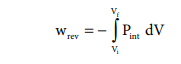Home | | Chemistry 11th std | Work involved in expansion and compression processes

# Work involved in expansion and compression processes

In most thermodynamic calculations we are dealing with the evaluation of work involved in the expansion or compression of gases.

Pressure - volume work

In elementary thermodynamics the only type of work generally considered is the work done in expansion (or compression) of a gas. This is known as pressure-volume work, PV work or expansion work.

## Work involved in expansion and compression processes:

In most thermodynamic calculations we are dealing with the evaluation of work involved in the expansion or compression of gases. The essential condition for expansion or compression of a system is that there should be difference between external pressure (Pext) and internal pressure (Pint).

For understanding pressure-volume work, let us consider a cylinder which contains 'n' moles of an ideal gas fitted with a frictionless piston of cross sectional area A. The total volume of the gas inside is Vi and pressure of the gas inside is Pint.

If the external pressure Pext is greater than Pint, the piston moves inward till the pressure inside becomes equal to Pext. Let this change be achieved in a single step and the final volume be Vf.

In this case, the work is done on the system (+w). It can be calculated as follows

w = ŌĆōF .Ōłåx ------------ (7.2)

where dx is the distance moved by the piston during the compression and F is the force acting on the gas.F= Pext A---- (7.3)

Substituting 7.3 in 7.2

w =ŌĆō Pext . A. Ōłåx

A. Ōłåx = change in volume = Vf - Vi

w =ŌĆō Pext . (Vf - Vi) -ŌĆōŌĆōŌĆōŌĆōŌĆō (7.4)

w =ŌĆō Pext . (ŌĆōŌłåV) ŌĆōŌĆōŌĆōŌĆōŌĆōŌĆō (7.5)

=Pext . ŌłåV

Since work is done on the system, it is a positive quantity.

If the pressure is not constant, but changes during the process such that it is always infinitesimally greater than the pressure of the gas, then, at each stage of compression, the volume decreases by an infinitesimal amount, dV. In such a case we can calculate the work done on the gas by the relationIn a compression process, Pext the external pressure is always greater than the pressure of the system.

i.e Pext = (Pint + dP).

In an expansion process, the external pressure is always less than the pressure of the system

i.e. Pext = (Pint - dP).When pressure is not constant and changes in infinitesimally small steps (reversible conditions) during compression from Vi to Vf , the P-V plot looks like in fig 7.4 Work done on the gas is represented by the shaded area.

In general case we can write,

Pext = (Pint + dP).

Such processes are called reversible processes. For a compression process work can be related to internal pressure of the system under reversible conditions by writing equationFor a given system with an ideal gas

Pint V = nRTIf Vf >Vi (expansion), the sign of work done by the process is negative.

If Vf <Vi (compression) the sign of work done on the process is positive.Study Material, Lecturing Notes, Assignment, Reference, Wiki description explanation, brief detail
11th Chemistry : UNIT 7 : Thermodynamics : Work involved in expansion and compression processes |